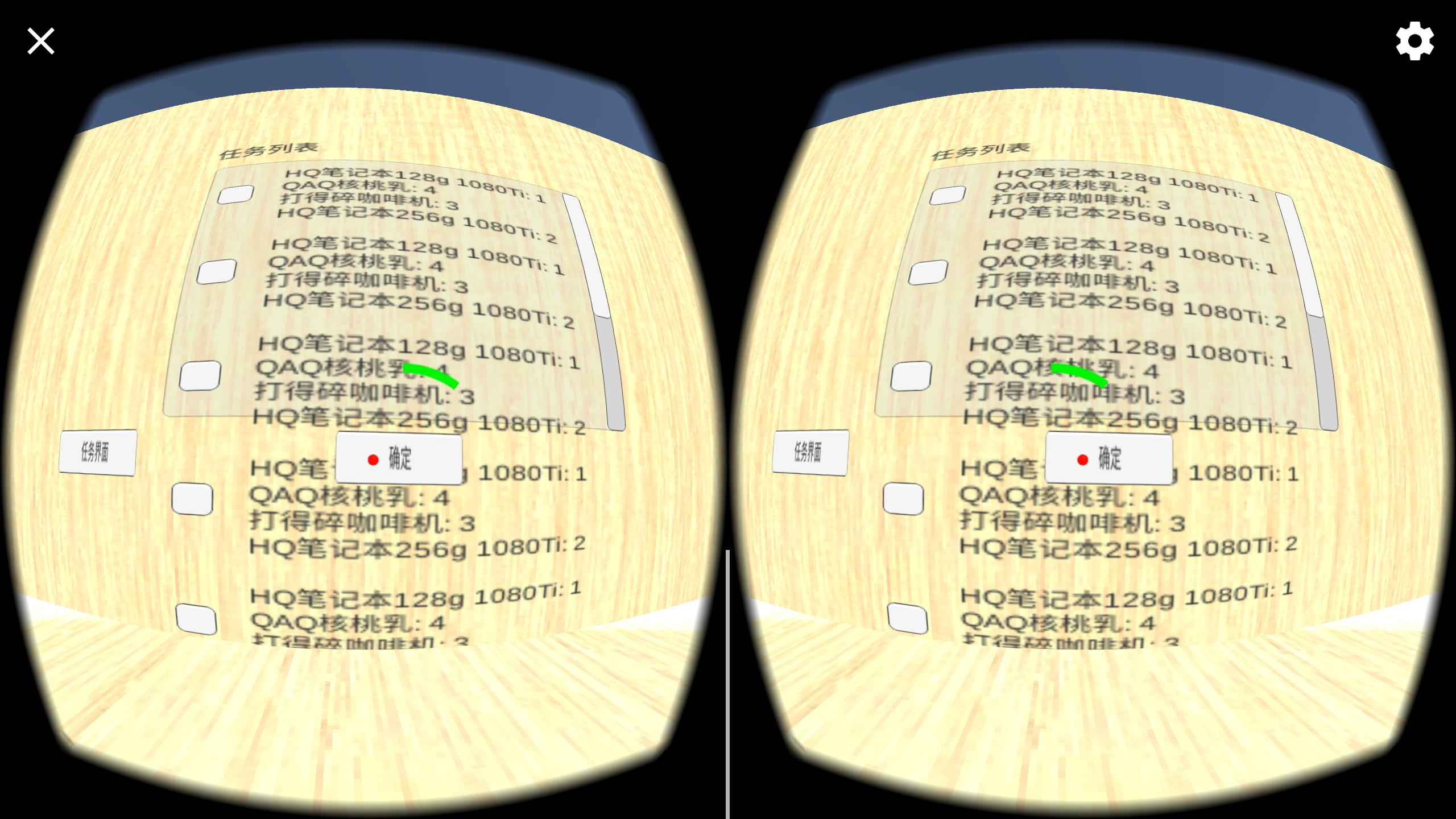UI显示正常UI显示没有遮挡效果

``````if (Physics.Raycast(ray, out hitInfo, 100F))
{
sp.transform.position = hitInfo.point;
//Debug.Log("UI层："+ results.Count);
if (hitInfo.transform.tag.Equals("货物"))
{
P_ring.GetComponent<Image>().color = Color.green;
{
hitInfo.transform.position = cao_p.transform.position;
P_ring.GetComponent<Image>().fillAmount = 0;

}
}
else if (hitInfo.transform.tag.Equals("地面"))
{
//P_ring.GetComponent<Image>().color = Color.grey;
{
P_ring.GetComponent<Image>().fillAmount = 0;

}
}
else if (hitInfo.transform.tag.Equals("标签"))
{
P_ring.GetComponent<Image>().color = Color.yellow;
//Debug.Log("标签");
{
hitInfo.transform.parent.parent.gameObject.GetComponent<货架>().setoff();
P_ring.GetComponent<Image>().fillAmount = 0;

}
}
else if (hitInfo.transform.tag.Equals("可触发"))
{
P_ring.GetComponent<Image>().color = Color.white;
//Debug.Log("可触发");
{
//hitInfo.transform.parent.parent.gameObject.GetComponent<货架>().setoff();
if (hitInfo.transform.name.Equals("Checkmark"))
{
hitInfo.transform.parent.parent.GetComponent<Toggle>().isOn = !hitInfo.transform.parent.parent.GetComponent<Toggle>().isOn;
}
else if (hitInfo.transform.name.EndsWith("按钮"))
{
hitInfo.transform.GetComponent<Button>().onClick.Invoke();
}
P_ring.GetComponent<Image>().fillAmount = 0;

}
}
else
{
P_ring.GetComponent<Image>().fillAmount = 0.1f;
}
}

``````

1. 射线在两种情况下都能检测到附加在UI组件上的盒型碰撞盒
2. 在USB调试或真机测试时不能检测碰撞盒所属gameobject的tag
3.在USB调试或真机测试时UI显示出现如图2所示问题